## Saturday, May 30, 2015

### Bug fix for ltt with node labels

Today, Luke Harmon & Josef Uyeda reported a bug in the phytools function `ltt`, which does lineage-through-time plots. The difference between `ltt` and equivalent functions in other packages is that `ltt` permits trees to include lineages that end prior to the end of the tree (for instance, a tree with some lineages that have gone extinct). For example:

``````library(phytools)
tree<-pbtree(n=100,b=1.6,d=0.6)
plotTree(tree,ftype="off")
``````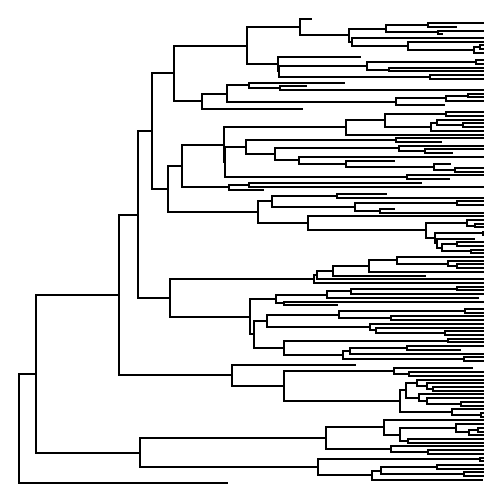``````obj<-ltt(tree)
``````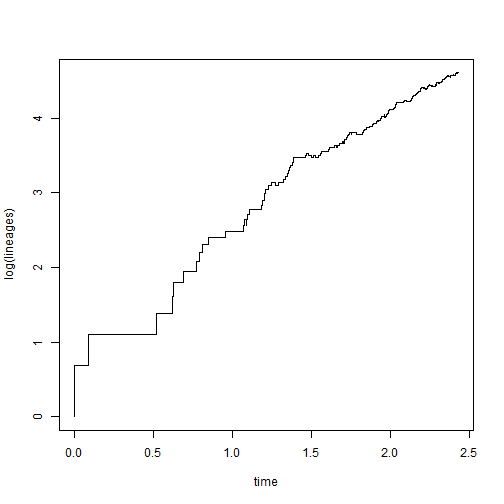The reported bug is for conditions when the tree includes node labels. The source of this issue is that for trees in which all tips are contemporaneous (i.e., ultrametric trees) `ltt` uses the ape function `branching.times` internally and assumes that the names of the vector returned by `branching.times` are the node indices from the `"phylo"` object. They are if the tree lacks node labels; however if node labels are present, then they will instead contain the node labels of the tree. This creates an error when `ltt` looks for the node indices & doesn't find them! Here's a demo:

``````tree<-pbtree(n=26,tip.label=LETTERS,scale=10)
plotTree(tree)
``````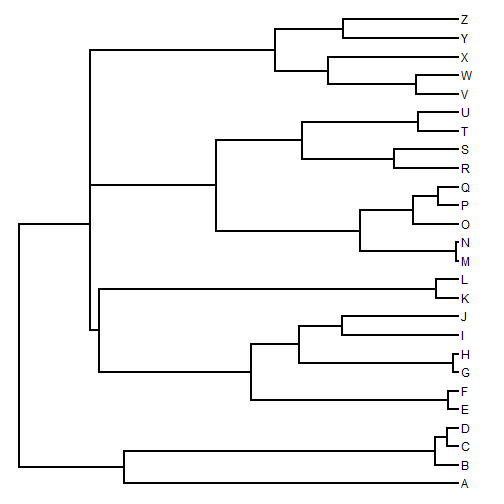``````## no node labels
ltt(tree)
``````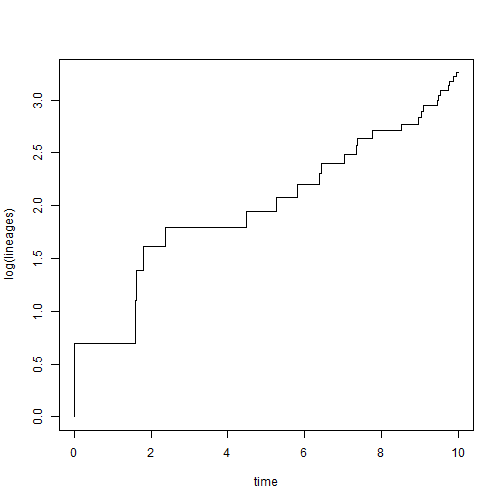``````## \$ltt
##    27 31 39 32 28 40 33 48 35 45 49 37 51 41 46 43 50 47 29 38 44 30 34 36
##  1  2  3  4  5  6  7  8  9 10 11 12 13 14 15 16 17 18 19 20 21 22 23 24 25
## 42
## 26 26
##
## \$times
##                        27           31           39           32
## 0.000000e+00 9.999983e-10 1.594837e+00 1.613968e+00 1.809402e+00
##           28           40           33           48           35
## 2.384807e+00 4.478021e+00 5.274344e+00 5.812117e+00 6.371105e+00
##           45           49           37           51           41
## 6.434691e+00 7.038966e+00 7.336978e+00 7.372788e+00 7.762271e+00
##           46           43           50           47           29
## 8.524592e+00 8.967709e+00 9.034180e+00 9.082955e+00 9.457377e+00
##           38           44           30           34           36
## 9.480731e+00 9.539301e+00 9.748171e+00 9.763473e+00 9.872351e+00
##           42
## 9.946383e+00 1.000000e+01
##
## \$gamma
##  0.8561059
##
## \$p
##  0.3919392
``````
``````## add node labels
tree\$node.label<-letters[1:25]
plotTree(tree)
nodelabels(tree\$node.label)
``````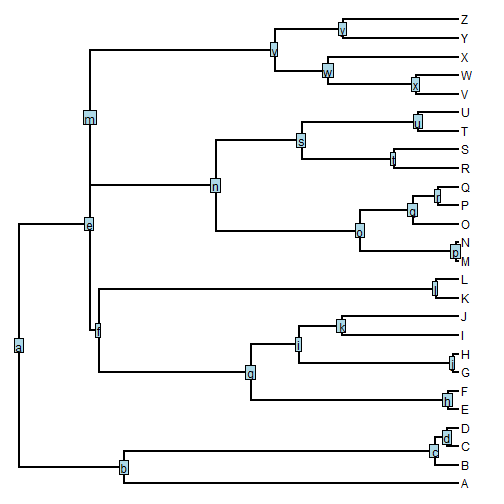``````ltt(tree)
``````
``````## Warning in ltt(tree): NAs introduced by coercion
``````
``````## Error in 2:n: NA/NaN argument
``````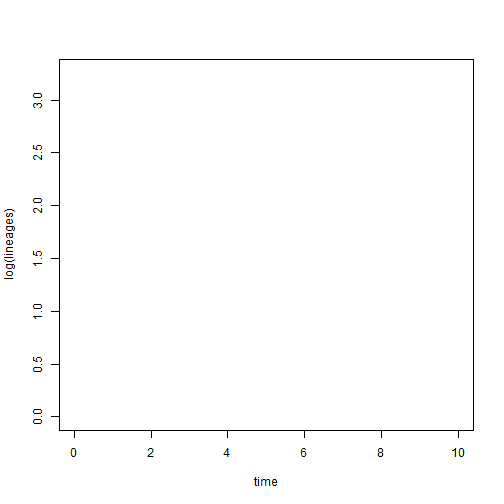My bug fix, which is a little hacky to be fair, I first copy the node labels from the tree into a vector with names corresponding to the node indices, then I strip them, then I replace the names in the object returned by `ltt` with the original labels. The code is here. The following is a demo in which I load the function from source:

``````source("http://www.phytools.org/ltt/v1.0/ltt.R")
ltt(tree)
````````````## \$ltt
##     a  e  m  f  b  n  g  v  i  s  w  k  y  o  t  q  x  u  c  l  r  d  h  j
##  1  2  3  4  5  6  7  8  9 10 11 12 13 14 15 16 17 18 19 20 21 22 23 24 25
##  p
## 26 26
##
## \$times
##                         a            e            m            f
## 0.000000e+00 9.999983e-10 1.594837e+00 1.613968e+00 1.809402e+00
##            b            n            g            v            i
## 2.384807e+00 4.478021e+00 5.274344e+00 5.812117e+00 6.371105e+00
##            s            w            k            y            o
## 6.434691e+00 7.038966e+00 7.336978e+00 7.372788e+00 7.762271e+00
##            t            q            x            u            c
## 8.524592e+00 8.967709e+00 9.034180e+00 9.082955e+00 9.457377e+00
##            l            r            d            h            j
## 9.480731e+00 9.539301e+00 9.748171e+00 9.763473e+00 9.872351e+00
##            p
## 9.946383e+00 1.000000e+01
##
## \$gamma
##  0.8561059
##
## \$p
##  0.3919392
``````

This update should be in the next version of phytools.

That's it.

## Friday, May 29, 2015

### Overlaying a tree on an LTT plot

I'm just in the middle of fixing a bug in the phytools function for lineage-through-time plots, `ltt`, pointed out to me by Luke Harmon. It has to do with node labels & is not that interesting (but I will nonetheless post the fixed version soon). However, in the process of playing around with that function, a discovered a find of neat visualization that involves overlaying a phylogeny on an LTT plot.

Here is a demo:

``````library(phytools)
## simulate tree
tree<-pbtree(n=26,scale=10) ## arbitrary
``````

The next part uses a function to automatically generate from a starting color a color with a certain `alpha` level of transparency. This function was posted to R-sig-phylo by Josef Uyeda:

``````makeTransparent<-function(someColor,alpha=10){
newColor<-col2rgb(someColor)
apply(newColor,2,function(curcoldata){
rgb(red=curcoldata,green=curcoldata,blue=curcoldata,
alpha=alpha,maxColorValue=255)
})
}
``````

Now we're ready to create our visualization:

``````obj<-ltt(tree)
mar=par()\$mar)
``````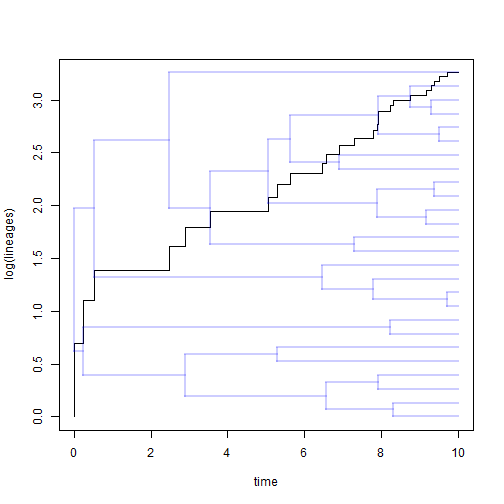We might even add other features - like vertical lines so we can see where lineages that have accumulated appear. This works well particularly for small trees:

``````tree<-pbtree(n=15,scale=10)
obj<-ltt(tree)
for(i in 3:(length(obj\$ltt)-1))
lines(rep(obj\$times[i],2),par()\$usr[3:4],lty="dashed",
col=makeTransparent("black",alpha=50))
mar=par()\$mar)
``````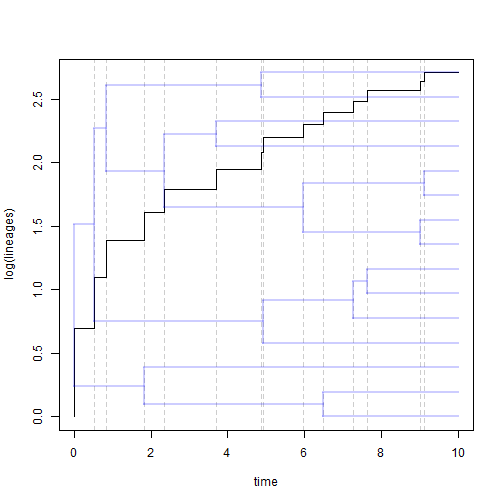That's all.

## Thursday, May 28, 2015

### Phylogenetic canonical correlation analysis using contrasts

phytools has a function, described in a paper by myself & Alexis Harrison, that does phylogenetic canonical correlation analysis in a phylogenetic context.

It is possible to do the same analysis, that is returning the same correlations & proportional coefficients, if we just first compute Felsenstein's contrasts, and then perform an uncentered canonical correlationa analysis on the contrasts. Here is a quick demo of how we go about doing this for data contained in matrices `X` and `Y`, and phylogeny `tree`.

``````library(phytools)

cca1<-cancor(apply(X,2,pic,phy=tree),apply(Y,2,pic,phy=tree),
xcenter=FALSE,ycenter=FALSE)
cca1\$cor
``````
``````##   0.9496915 0.9099798 0.8602210 0.8278812 0.8035111 0.7906251 0.7601731
##   0.7348214 0.6872831 0.5698925 0.5605435 0.4835210 0.4116497 0.2441289
``````
``````cca2<-phyl.cca(tree,X,Y)
cca2\$cor
``````
``````##   0.9496915 0.9099798 0.8602210 0.8278812 0.8035111 0.7906251 0.7601731
##   0.7348214 0.6872831 0.5698925 0.5605435 0.4835210 0.4116497 0.2441289
``````

Just to verify that the correlations are the same and that the coefficients are proportional, let's quickly plot them:

``````## this is 1:1
plot(cca1\$cor,cca2\$cor)
``````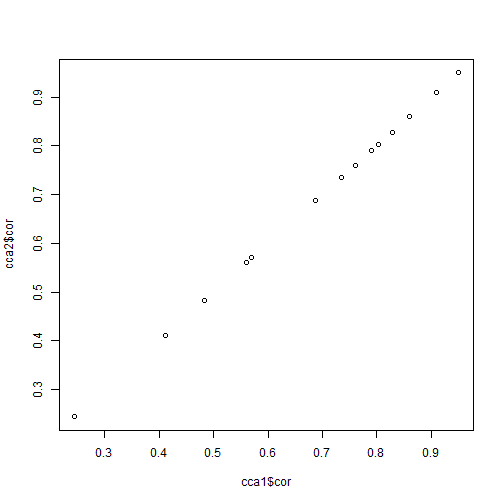``````## this is 1:1 or 1:-1, because the sign of the coefficients for
## any canonical axis is arbitrary
## (in addition, be aware that the scale between phylogenetic &
## non-phylogenetic analyses will probably differ)
plot(cca1\$xcoef,cca2\$xcoef)
``````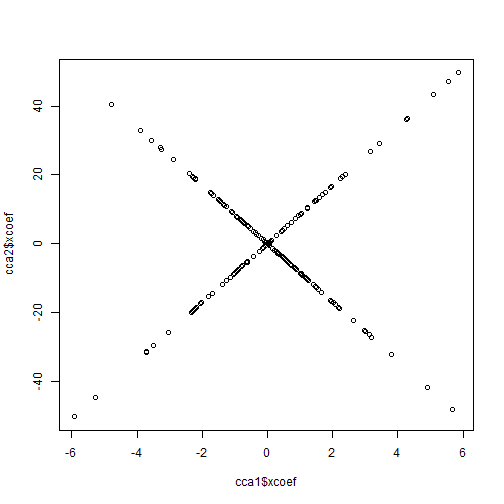The main advantage of `phyl.cca` is that it also returns scores in the original space, and it automatically runs hypothesis tests on the canonical correlations. There are other canonical correlation functions to do this in R, but they do not permit the data to be treated as centered, which means that they cannot be used with contrasts.

This post is based on a user query about how to do this, by the way.

That's all there is to it.